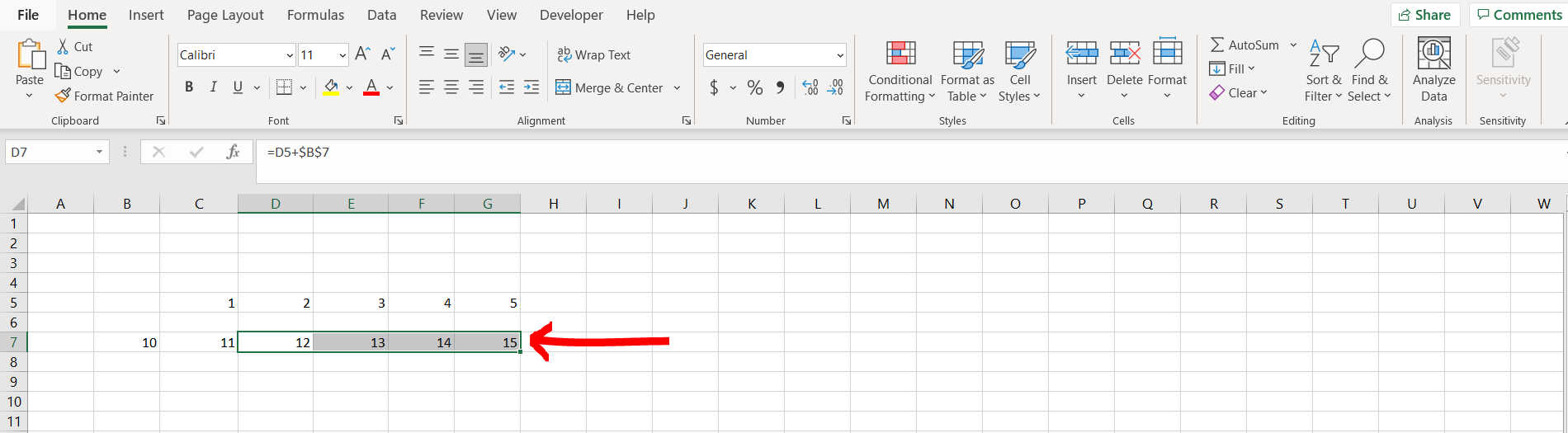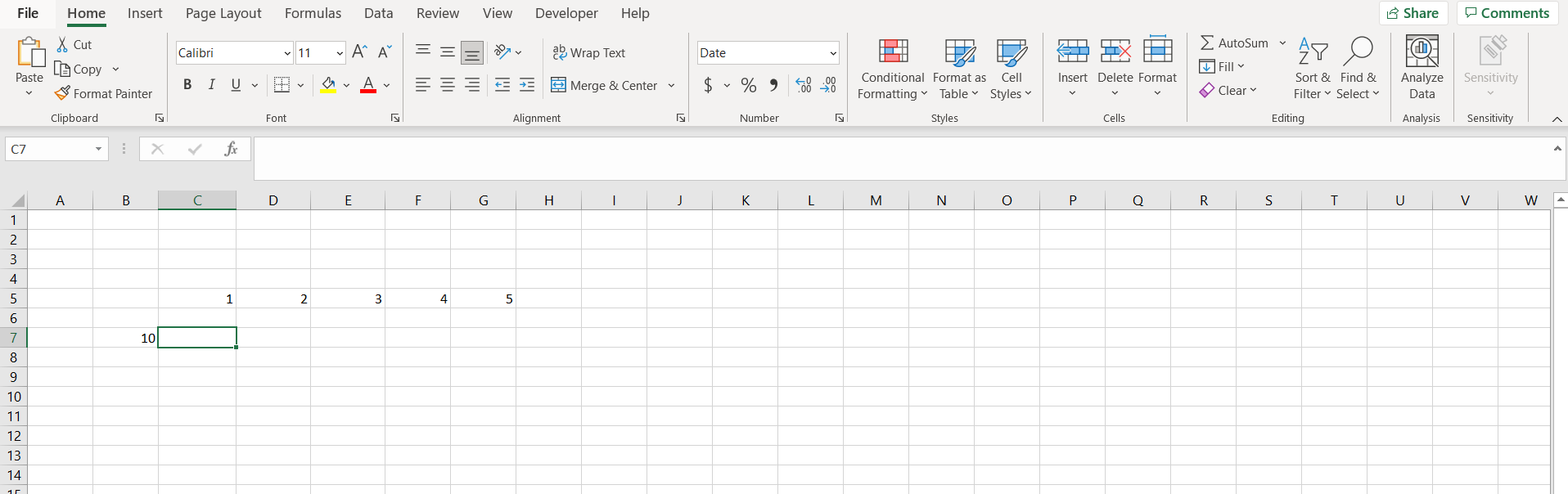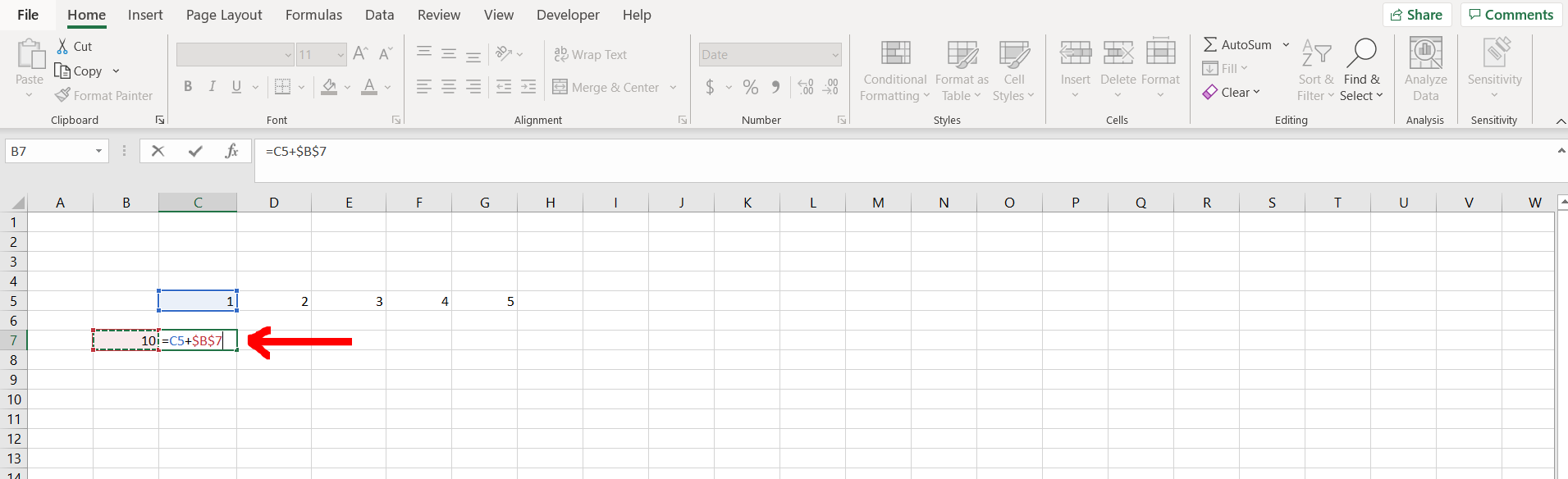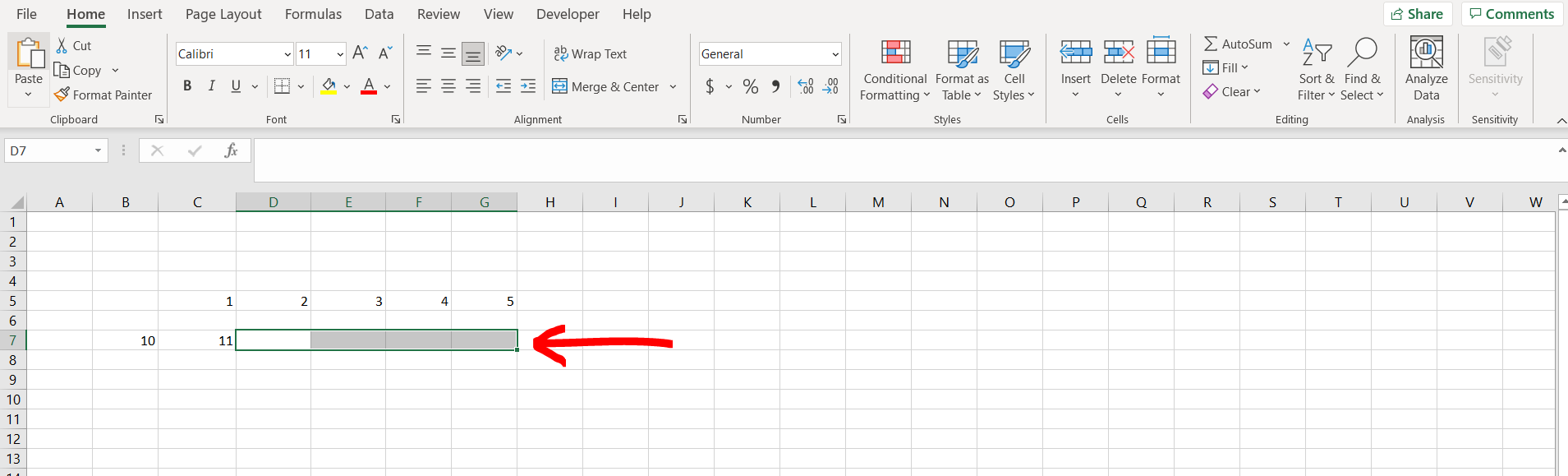# How to keep one cell constant in ExcelExcel offers a very interesting way to keep one cell constant. We can cater to this problem statement by using the “F4” key. We can perform the below mentioned way to keep one cellr constant in excel:

### Step 1 – Excel workbook with some cells with numbers– Open the desired Excel workbook containing some cells with numbers

### Step 2 – Using the F4 keys– Now click in the cell where you want to add the formula, then while referring to the cell containing the number which you want to keep constant, press “F4” after giving reference to this cell, and then hit Enter. For example we want to add each number in row 5 with the number in cell “B7”, hence while using cell “B7”, we have pressed F4. We can see that post pressing F4 “B7” has become “\$B\$7”. This means that the cell with the number has been kept constant

### Step 3 – Copying the formula– Now copy the formula typed in this cell and paste it across all the other cells as shown in the image above

### Step 4 – Formula applied– We can see that while pasting the formula to other cells, the constant cell number did not change, and hence we could add all the numbers to the fixed cell as shown in the image above